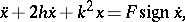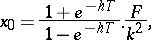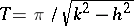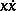# Auto-oscillation

A non-damped oscillation in a non-linear dynamical system, whose amplitude and frequency can remain constant during a long period of time, are largely independent of the initial conditions, and are determined by the properties of the system itself (see also Oscillations, theory of). The term "auto-oscillation" was introduced by A.A. Andronov (see ).

Dynamical systems capable of performing oscillations are known as auto-oscillating systems. They include clocks, generators of electric vibrations, electric bells, blow and bow musical instruments, etc. Under certain conditions auto-oscillations can also be produced in dynamical systems which normally function without auto-oscillations (e.g. auto-oscillations in the front suspension of a car- "noise" , the flutter of an aircraft wing, or auto-oscillations in automatic control and monitoring systems). The simplest auto-oscillating system can be represented as consisting of a constant source of energy, a mechanism regulating the supply of energy to the oscillating system, and the oscillating system itself. An essential feature of such a system is its feedback nature: on the one hand, the regulating mechanism controls the motion of the oscillating system but, on the other, it is the motion of the oscillating system that influences the operation of the regulating mechanism . From the mathematical point of view, autonomous auto-oscillating systems with one degree of freedom may be defined as systems whose equations of motion have one or more limit cycles in the phase plane (cf. Limit cycle).

An important typical property of auto-oscillations is that their amplitude is largely independent of the initial conditions, i.e. the existence of one or more ranges of initial conditions such that to any initial set of conditions within any one of these ranges there corresponds the same auto-oscillation amplitude. This means that oscillating systems may contain several stationary processes with different amplitudes; each one of these becomes realized in the system, depending on a choice of a region of initial conditions. This property constitutes the basic difference between periodic motion in an auto-oscillating system and periodic motion in a conservative system. Another typical property of auto-oscillating systems is the fact that the periods of the auto-oscillations are determined by the properties of the system itself and are not imposed from outside. This is the basic difference between auto-oscillations and forced oscillations.

An essential feature of auto-oscillations consists in the fact that the loss of energy must be compensated by a constant source of energy. Since in an autonomous system the forces do not explicitly depend on time, this source of energy must produce a force which is not a given function of time and which is determined by the system itself. Constant sources of energy include the winding mechanism in a clock, a shaft rotating at a constant angular velocity, an endless belt moving at a constant rate, or a jet of liquid or gas having a constant velocity; in systems in which the motive force is an electric flow, an electric battery may serve as such a source.

An example of a very simple auto-oscillating system is a pendulum in a viscous frictional medium, acted upon by a force of constant magnitude which always acts in the direction of the motion. The differential equation of this dynamical system iswhereare constant coefficients. Under any initial conditions a periodic oscillation becomes established in the system, the maximum deflection of the pendulum from its position of equilibrium (amplitude) beingwhereis the half-period of the periodic motion. A stable limit cycle in the phase planecorresponds to this motion .

A typical property of all auto-oscillating systems is the connection between the constant source of energy and the system, which is such that the energy given by the source varies periodically, the period of this variation being determined by the properties of the system. This may be expressed by saying that an auto-oscillating system is a system which executes a periodic process at the expense of a non-periodic energy source. The shape of auto-oscillations can be close to sinusoidal, but can also be very different. The latter type of auto-oscillations is known as relaxation oscillation. Auto-oscillations with a shape close to sinusoidal usually occur in systems in which the loss in energy during one period is small, and consequently, the amount of the energy fed into the system is also small. They may also occur when the energy losses in the system during one period are large, but if the parameters are properly chosen, the losses are compensated not merely for each period, but also for each small fraction of a period. Such systems include the so-called RC-generators of sinusoidal oscillations . During relaxation oscillations energy losses are usually large and are compensated for after one period by almost the entire energy of the oscillating system.

The generation of auto-oscillations may be "soft" or "hard" . In the former case, variation of some parameter of a system in a stable equilibrium results in the generation of auto-oscillations whose amplitude steadily increases from zero as the parameter is continuously varied. If the parameter is now varied in the opposite direction, the amplitude of the auto-oscillation steadily decreases to zero, and the system returns to a stable equilibrium. If the generation of the auto-oscillations is hard, the system passes from a state of stable equilibrium to an auto-oscillation with finite amplitude as one of its parameters is slowly and continuously varied; as the parameter is further varied, the rate of increase of the amplitude becomes constant. If the parameter is varied in the reverse direction, the amplitude continuously decreases and, after it has attained a certain value, the system returns to the state of stable equilibrium. It is important to note in this connection that the system passes from stable equilibrium to auto-oscillations and from auto-oscillations back to stable equilibrium at different values of the parameter.

Auto-oscillating systems display an interesting and important property — the effect of enforced synchronization, which is sometimes called "capturecapture" or "mode lockingmode locking" . This takes place when the difference between the frequency of the auto-oscillating system and that of the external force acting on it is sufficiently small. The steady periodic motion of the system then assumes the frequency of the external force, i.e. the external force imparts its own frequency to the auto-oscillating system , .